io7m | single-page | multi-page | archive (zip, signature)
A Brief History Of Vertex Specification In OpenGL
Overview
Graphics hardware has rapidly evolved from simple accelerated framebuffer devices up to modern day general purpose GPUs with programmable pipelines. As such, the OpenGL API that first appeared in 1992 encourages a programming style that works very poorly (from a performance perspective) on modern hardware. Subsequent revisions of the API have steadily removed obsolete methods of programming to focus on providing a small and simple interface to the extremely general programming model that modern graphics hardware allows.
This document attempts to describe the different vertex specification methods that have been added to OpenGL over the course of its development.
Immediate mode
Around the mid-1990s, 3D graphics hardware was appearing in consumer grade desktop PCs, workstations, and game consoles. OpenGL, at that time, appeared as a simple "immediate" API. The word "immediate" refers to the imperative nature of the API where the user programs a sequence of translation, rotation, and drawing commands. As an example, the user might call the following function once per frame to draw a triangle onscreen:
```void
draw_triangle(void)
{
glPushMatrix();
glTranslated(20.0, 20.0, 0.0);
glBegin(GL_TRIANGLES);
glColor3d(1.0, 0.0, 0.0);
glVertex3d(0.0, 0.0, 0.0);
glColor3d(0.0, 1.0, 0.0);
glVertex3d(1.0, 0.0, 0.0);
glColor3d(0.0, 0.0, 1.0);
glVertex3d(1.0, 1.0, 0.0);
glEnd();
glPopMatrix();
}
```
The above code would likely result in an image similar to the following:The API modelled the graphics card as a large state machine with dozens of variables (such as "the current colour", modified with glColor3d()). It also required graphics hardware to implement multiple stacks of matrices to control projection, object orientation, object scaling, amongst other things.
As hardware evolved, it quickly became clear that the immediate mode model was hard to optimize for at the driver level. Programmers could submit vertex data and state changes in any arbitrary order and the drivers could make very few assumptions about the immutability of state at any given time. The immediate mode model was also grossly inefficient in terms of how vertex data was actually specified: real 3D mesh data requires much more than just simple vertex coordinates. A typical 3D mesh might require a colour value (glColor()), a normal vector (glNormal()), multiple texture coordinate values (glTexCoord()), fog coordinates (glFogCoord()), and more. This results in a huge number of function calls, even for relatively small models.
A complete example is as follows (source):
```#include <assert.h>
#include <GL/glut.h>
#include <GL/glext.h>

static void
reshape(int width, int height)
{
glViewport(0, 0, width, height);

glMatrixMode(GL_PROJECTION);
glOrtho(0, width, 0, height, -1.0, 100.0);

assert(glGetError() == GL_NO_ERROR);
}

static void
display(void)
{
glClearColor(0.3f, 0.3f, 0.3f, 1.0f);
glClear(GL_COLOR_BUFFER_BIT);

glMatrixMode(GL_MODELVIEW);

glPushMatrix();
glTranslated(20.0, 20.0, 0.0);
glBegin(GL_TRIANGLES);
glColor3d(100.0, 0.0, 0.0);
glVertex3d(0.0, 0.0, 0.0);
glColor3d(0.0, 100.0, 0.0);
glVertex3d(100.0, 0.0, 0.0);
glColor3d(0.0, 0.0, 100.0);
glVertex3d(100.0, 100.0, 0.0);
glEnd();
glPopMatrix();

glutSwapBuffers();
}

int
main(int argc, char **argv)
{
glutInit(&argc, argv);
glutCreateWindow("Immediate mode triangle");
glutReshapeFunc(reshape);
glutDisplayFunc(display);
glutIdleFunc(glutPostRedisplay);

glutMainLoop();
return 0;
}
```
Display Lists
Display lists were a means of compiling a list of OpenGL instructions and executing them at a later date. They were arguably not a means of vertex specification, specifically (pun intended) but were often used to improve the speed of doing so. They are covered here for the sake of completeness.
A complete example is as follows (source):
```#include <assert.h>
#include <GL/glut.h>
#include <GL/glext.h>

static int list;

static void
reshape(int width, int height)
{
glViewport(0, 0, width, height);

glMatrixMode(GL_PROJECTION);
glOrtho(0, width, 0, height, -1.0, 100.0);

assert(glGetError() == GL_NO_ERROR);
}

static void
init(void)
{
list = glGenLists(1);

glNewList(list, GL_COMPILE);
glBegin(GL_TRIANGLES);
glColor3d(100.0, 0.0, 0.0);
glVertex3d(0.0, 0.0, 0.0);
glColor3d(0.0, 100.0, 0.0);
glVertex3d(100.0, 0.0, 0.0);
glColor3d(0.0, 0.0, 100.0);
glVertex3d(100.0, 100.0, 0.0);
glEnd();
glEndList();
}

static void
display(void)
{
glClearColor(0.3f, 0.3f, 0.3f, 1.0f);
glClear(GL_COLOR_BUFFER_BIT);

glMatrixMode(GL_MODELVIEW);

glPushMatrix();
glTranslated(20.0, 20.0, 0.0);
glCallList(list);
glTranslated(120.0, 120.0, 0.0);
glCallList(list);
glPopMatrix();

glutSwapBuffers();
}

int
main(int argc, char **argv)
{
glutInit(&argc, argv);
glutCreateWindow("Display list triangle");
init();
glutReshapeFunc(reshape);
glutDisplayFunc(display);
glutIdleFunc(glutPostRedisplay);

glutMainLoop();
return 0;
}

```
As can be seen from the output, the triangle drawing commands are compiled into a display list and then called twice to draw two triangles. The commands in the display list were typically stored in the OpenGL implementation's memory in order to reduce the number of commands sent between the program and the OpenGL driver. Display lists were obviously not a solution to the general inefficiencies of the immediate mode.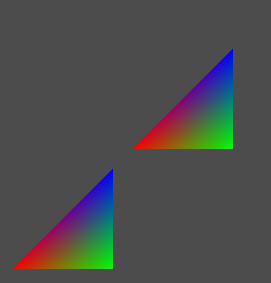Vertex Arrays
Vertex arrays first appeared in OpenGL 1.1 as a means to more efficiently specify vertex data. The programmer allocates an array in ordinary system memory (using standard C malloc(), for example), fills it with vertex data and then calls one function to draw vertices using that array as the data source. As an example (source):
```#include <assert.h>
#include <GL/glut.h>
#include <GL/glext.h>

typedef float vector3;

static vector3 vertices[] = {
{ 0.0,   0.0,   0.0 },
{ 100.0, 0.0,   0.0 },
{ 100.0, 100.0, 0.0 }
};

static void
reshape(int width, int height)
{
glViewport(0, 0, width, height);

glMatrixMode(GL_PROJECTION);
glOrtho(0, width, 0, height, -1.0, 100.0);

assert(glGetError() == GL_NO_ERROR);
}

static void
display(void)
{
glClearColor(0.3f, 0.3f, 0.3f, 1.0f);
glClear(GL_COLOR_BUFFER_BIT);

glMatrixMode(GL_MODELVIEW);

glPushMatrix();
glTranslated(20.0, 20.0, 0.0);
glEnableClientState(GL_VERTEX_ARRAY);
glVertexPointer(3, GL_FLOAT, 0, vertices);
glDrawArrays(GL_TRIANGLES, 0, 3);
glDisableClientState(GL_VERTEX_ARRAY);
glPopMatrix();

glutSwapBuffers();
}

int
main(int argc, char **argv)
{
glutInit(&argc, argv);
glutCreateWindow("Vertex array triangle");
glutReshapeFunc(reshape);
glutDisplayFunc(display);
glutIdleFunc(glutPostRedisplay);

glutMainLoop();
return 0;
}
```
The glEnableClientState(GL_VERTEX_ARRAY) call enables the use of client-side vertex arrays. The glVertexPointer(3, GL_FLOAT, 0, vertices) call tells OpenGL that the array referenced by vertices contains three-element vertices of type GL_FLOAT, with 0 bytes between each successive element. The glDrawArrays(GL_TRIANGLES, 0, 3) call asks OpenGL to draw three vertices, starting at offset 0.The API allows the programmer quite a lot of freedom in terms of how the data is arranged in order to be passed to OpenGL. The following example shows the storing of vertex coordinates and colour values in two separate arrays (source):
```#include <assert.h>
#include <GL/glut.h>
#include <GL/glext.h>

typedef float vector3;

static vector3 vertices[] = {
{ 0.0,   0.0,   0.0 },
{ 100.0, 0.0,   0.0 },
{ 100.0, 100.0, 0.0 }
};
static vector3 colors[] = {
{ 1.0, 0.0, 0.0 },
{ 0.0, 1.0, 0.0 },
{ 0.0, 0.0, 1.0 }
};

static void
reshape(int width, int height)
{
glViewport(0, 0, width, height);

glMatrixMode(GL_PROJECTION);
glOrtho(0, width, 0, height, -1.0, 100.0);

assert(glGetError() == GL_NO_ERROR);
}

static void
display(void)
{
glClearColor(0.3f, 0.3f, 0.3f, 1.0f);
glClear(GL_COLOR_BUFFER_BIT);

glMatrixMode(GL_MODELVIEW);

glPushMatrix();
glTranslated(20.0, 20.0, 0.0);

glEnableClientState(GL_VERTEX_ARRAY);
glEnableClientState(GL_COLOR_ARRAY);

glVertexPointer(3, GL_FLOAT, 0, vertices);
glColorPointer(3, GL_FLOAT, 0, colors);
glDrawArrays(GL_TRIANGLES, 0, 3);

glDisableClientState(GL_VERTEX_ARRAY);
glDisableClientState(GL_COLOR_ARRAY);

glPopMatrix();

assert(glGetError() == GL_NO_ERROR);

glutSwapBuffers();
}

int
main(int argc, char **argv)
{
glutInit(&argc, argv);
glutCreateWindow("Vertex array triangle");
glutReshapeFunc(reshape);
glutDisplayFunc(display);
glutIdleFunc(glutPostRedisplay);

glutMainLoop();
return 0;
}
```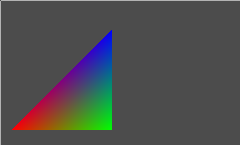The above example uses separate arrays for each vertex attribute. For better cache locality, it is preferable to store one array of a record type and then tell OpenGL how to retrieve the values it needs from this single array. An example of this is as follows (source):
```#include <assert.h>
#include <stddef.h>
#include <GL/glut.h>
#include <GL/glext.h>

typedef float vector3;

/*
* Interleaved vertex and colour data.
*/

typedef struct
{
vector3 position;
vector3 colour;
} vertex;

static vertex data0[] = {
{ { 0.0,   0.0,   0.0 }, { 1.0, 0.0, 0.0 } },
{ { 100.0, 0.0,   0.0 }, { 0.0, 1.0, 0.0 } },
{ { 100.0, 100.0, 0.0 }, { 0.0, 0.0, 1.0 } }
};

/*
* Vertex data followed by colour data.
*/

static vector3 data1[] = {
{ 0.0,   0.0,   0.0 },
{ 100.0, 0.0,   0.0 },
{ 100.0, 100.0, 0.0 },

{ 1.0, 0.0, 0.0 },
{ 0.0, 1.0, 0.0 },
{ 0.0, 0.0, 1.0 }
};

static void
reshape(int width, int height)
{
glViewport(0, 0, width, height);

glMatrixMode(GL_PROJECTION);
glOrtho(0, width, 0, height, -1.0, 100.0);

assert(glGetError() == GL_NO_ERROR);
}

static void
display(void)
{
glClearColor(0.3f, 0.3f, 0.3f, 1.0f);
glClear(GL_COLOR_BUFFER_BIT);

glMatrixMode(GL_MODELVIEW);

glPushMatrix();
glTranslated(20.0, 20.0, 0.0);

{
const unsigned char *data0_v = (unsigned char *) &data0;
const unsigned char *data0_c = ((unsigned char *) &data0) + offsetof(vertex, colour);

glEnableClientState(GL_VERTEX_ARRAY);
glEnableClientState(GL_COLOR_ARRAY);
glVertexPointer(3, GL_FLOAT, sizeof(vertex), data0_v);
glColorPointer(3, GL_FLOAT, sizeof(vertex), data0_c);
glDrawArrays(GL_TRIANGLES, 0, 3);
glDisableClientState(GL_VERTEX_ARRAY);
glDisableClientState(GL_COLOR_ARRAY);
}

glTranslated(120.0, 120.0, 0.0);

{
const unsigned char *data1_v = (unsigned char *) &data1;
const unsigned char *data1_c = ((unsigned char *) &data1) + (3 * sizeof(vector3));

glEnableClientState(GL_VERTEX_ARRAY);
glEnableClientState(GL_COLOR_ARRAY);
glVertexPointer(3, GL_FLOAT, sizeof(vector3), data1_v);
glColorPointer(3, GL_FLOAT, sizeof(vector3), data1_c);
glDrawArrays(GL_TRIANGLES, 0, 3);
glDisableClientState(GL_VERTEX_ARRAY);
}

glPopMatrix();

assert(glGetError() == GL_NO_ERROR);

glutSwapBuffers();
}

int
main(int argc, char **argv)
{
glutInit(&argc, argv);
glutCreateWindow("Vertex array triangle");
glutReshapeFunc(reshape);
glutDisplayFunc(display);
glutIdleFunc(glutPostRedisplay);

glutMainLoop();
return 0;
}
```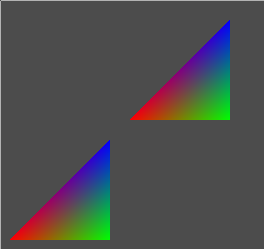Each glVertexPointer and glColorPointer call specifies a "stride" value and an initial offset into the array. The "stride" value specifies the number of bytes that need to be skipped in order to retrieve the next value of a given type from the array.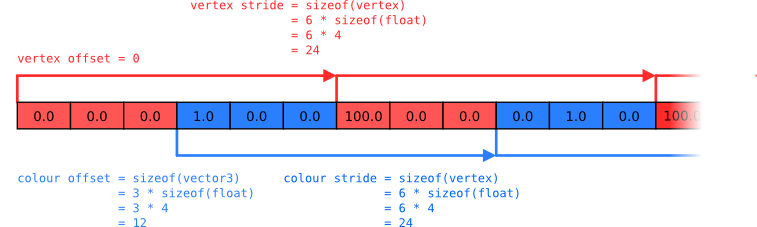As the illustration above shows: To read colour values, start at &data0 + offsetof(vertex, colour), read 3 values of type GL_FLOAT and then skip sizeof(vertex) bytes. Reading vertex position values is the same, except that the initial offset is 0 (reading directly from the start of the array). Obviously, an infinite number of permutations are possible. It is also possible to interleave other data into the array using functions such as glNormalPointer, glTexCoordPointer, etc.
It is also possible to specify an array of indices to be used for rendering, as opposed to having glDrawArrays simply walk through the current arrays (source):
```#include <assert.h>
#include <stddef.h>
#include <GL/glut.h>
#include <GL/glext.h>

typedef float vector3;

typedef struct
{
vector3 position;
vector3 colour;
} vertex;

static vertex data[] = {
{ { 0.0,   0.0,   0.0 }, { 1.0, 0.0, 0.0 } },
{ { 100.0, 0.0,   0.0 }, { 0.0, 1.0, 0.0 } },
{ { 100.0, 100.0, 0.0 }, { 0.0, 0.0, 1.0 } },
{ { 0.0,   100.0, 0.0 }, { 1.0, 1.0, 0.0 } },
};

static void
reshape(int width, int height)
{
glViewport(0, 0, width, height);

glMatrixMode(GL_PROJECTION);
glOrtho(0, width, 0, height, -1.0, 100.0);

assert(glGetError() == GL_NO_ERROR);
}

static void
display(void)
{
glClearColor(0.3f, 0.3f, 0.3f, 1.0f);
glClear(GL_COLOR_BUFFER_BIT);

glMatrixMode(GL_MODELVIEW);

glPushMatrix();
glTranslated(20.0, 20.0, 0.0);

glEnableClientState(GL_VERTEX_ARRAY);
glEnableClientState(GL_COLOR_ARRAY);

{
const unsigned char *data_v = (unsigned char *) &data;
const unsigned char *data_c = ((unsigned char *) &data) + offsetof(vertex, colour);

{
const unsigned char indices[] = { 0, 1, 2 };
glVertexPointer(3, GL_FLOAT, sizeof(vertex), data_v);
glColorPointer(3, GL_FLOAT, sizeof(vertex), data_c);
glDrawElements(GL_TRIANGLES, 3, GL_UNSIGNED_BYTE, indices);
}

glTranslated(120.0, 120.0, 0.0);

{
const unsigned char indices[] = { 1, 2, 3 };
glVertexPointer(3, GL_FLOAT, sizeof(vertex), data_v);
glColorPointer(3, GL_FLOAT, sizeof(vertex), data_c);
glDrawElements(GL_TRIANGLES, 3, GL_UNSIGNED_BYTE, indices);
}
}

glDisableClientState(GL_VERTEX_ARRAY);
glDisableClientState(GL_COLOR_ARRAY);

glPopMatrix();

assert(glGetError() == GL_NO_ERROR);

glutSwapBuffers();
}

int
main(int argc, char **argv)
{
glutInit(&argc, argv);
glutCreateWindow("Vertex array triangle");
glutReshapeFunc(reshape);
glutDisplayFunc(display);
glutIdleFunc(glutPostRedisplay);

glutMainLoop();
return 0;
}
```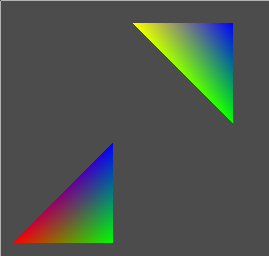The only new code is the use of glDrawElements, which takes an array of indices. Note that four vertices are declared in the vertex array and then glDrawElements is used to draw one triangle from vertices {0, 1, 2} and another triangle from vertices {1, 2, 3}.
Vertex Buffer Objects
Whilst vertex arrays removed the large number of function calls that were required per vertex under immediate mode, the problem remained that large amounts of vertex data had to be sent from the program to the OpenGL implementation each frame. If this data consisted entirely of static models (as is the case for many 3D applications), removing this repeated transmission of data would increase efficiency by orders of magnitude. The API already had functions that facilitated one-time bulk uploads of data: The glTexImage family, promoted from the EXT_texture_object extension in OpenGL 1.1. The programmer performs the following steps in order to upload a texture to OpenGL:
1. Obtain a new texture name/index with glGenTextures().
2. "Bind" the resulting name with glBindTexture(), making it the current texture.
3. Supply texture data as an array of bytes (or other formats) with glTexImage().
Later in the program, when actually drawing primitives, the programmer once again calls glBindTexture() to select a texture and then supplies texture coordinates to draw textured polygons. Note that at this point, the actual texture bitmap data could have been discarded by the program and may only exist in the OpenGL implementation's memory (most likely hardware texture memory).
In OpenGL 1.5, Vertex Buffer Objects (VBOs) were added, allowing vertex data to be uploaded to the OpenGL implementation and then possibly discarded from the program's memory space. Somewhat confusingly, the designers reused much of the Vertex Array API but added explicitly bound "buffers" in a similar manner to the texture API. As an example (source):
```#define GL_GLEXT_PROTOTYPES 1

#include <assert.h>
#include <stddef.h>
#include <stdlib.h>
#include <GL/glut.h>
#include <GL/glext.h>

typedef float vector3;

typedef struct
{
vector3 position;
vector3 colour;
} vertex;

static GLuint vertices_name;
static GLuint triangles;

static void
init(void)
{
vertex *data = malloc(4 * sizeof(vertex));
if (data == NULL) abort();

data.position = 0.0;
data.position = 0.0;
data.position = 0.0;
data.colour = 1.0;
data.colour = 0.0;
data.colour = 0.0;

data.position = 100.0;
data.position = 0.0;
data.position = 0.0;
data.colour = 0.0;
data.colour = 1.0;
data.colour = 0.0;

data.position = 100.0;
data.position = 100.0;
data.position = 0.0;
data.colour = 0.0;
data.colour = 0.0;
data.colour = 1.0;

data.position = 0.0;
data.position = 100.0;
data.position = 0.0;
data.colour = 1.0;
data.colour = 1.0;
data.colour = 0.0;

glGenBuffers(1, &vertices_name);
glBindBuffer(GL_ARRAY_BUFFER, vertices_name);
glBufferData(GL_ARRAY_BUFFER, 4 * sizeof(vertex), data, GL_STATIC_DRAW);

free(data);

glGenBuffers(2, triangles);

{
unsigned char indices[] = { 0, 1, 2 };
glBindBuffer(GL_ELEMENT_ARRAY_BUFFER, triangles);
glBufferData(GL_ELEMENT_ARRAY_BUFFER, sizeof(indices), indices, GL_STATIC_DRAW);
}

{
unsigned char indices[] = { 1, 2, 3 };
glBindBuffer(GL_ELEMENT_ARRAY_BUFFER, triangles);
glBufferData(GL_ELEMENT_ARRAY_BUFFER, sizeof(indices), indices, GL_STATIC_DRAW);
}

assert(glGetError() == GL_NO_ERROR);
}

static void
reshape(int width, int height)
{
glViewport(0, 0, width, height);

glMatrixMode(GL_PROJECTION);
glOrtho(0, width, 0, height, -1.0, 100.0);

assert(glGetError() == GL_NO_ERROR);
}

static void
display(void)
{
glClearColor(0.3f, 0.3f, 0.3f, 1.0f);
glClear(GL_COLOR_BUFFER_BIT);

glMatrixMode(GL_MODELVIEW);

glPushMatrix();
glTranslated(20.0, 20.0, 0.0);

glEnableClientState(GL_VERTEX_ARRAY);
glEnableClientState(GL_COLOR_ARRAY);

glBindBuffer(GL_ARRAY_BUFFER, vertices_name);
glBindBuffer(GL_ELEMENT_ARRAY_BUFFER, triangles);
glVertexPointer(3, GL_FLOAT, sizeof(vertex), (void *) 0);
glColorPointer(3, GL_FLOAT, sizeof(vertex), (void *) offsetof(vertex, colour));
glDrawElements(GL_TRIANGLES, 3, GL_UNSIGNED_BYTE, (void *) 0);

glTranslated(120.0, 120.0, 0.0);

glBindBuffer(GL_ARRAY_BUFFER, vertices_name);
glBindBuffer(GL_ELEMENT_ARRAY_BUFFER, triangles);
glVertexPointer(3, GL_FLOAT, sizeof(vertex), (void *) 0);
glColorPointer(3, GL_FLOAT, sizeof(vertex), (void *) offsetof(vertex, colour));
glDrawElements(GL_TRIANGLES, 3, GL_UNSIGNED_BYTE, (void *) 0);

glDisableClientState(GL_VERTEX_ARRAY);
glDisableClientState(GL_COLOR_ARRAY);

glPopMatrix();

assert(glGetError() == GL_NO_ERROR);

glutSwapBuffers();
}

int
main(int argc, char **argv)
{
glutInit(&argc, argv);
glutCreateWindow("Vertex array triangle");
init();
glutReshapeFunc(reshape);
glutDisplayFunc(display);
glutIdleFunc(glutPostRedisplay);

glutMainLoop();
return 0;
}

```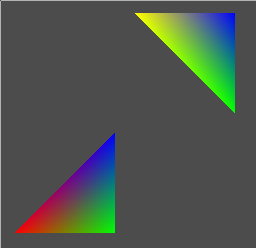Note that the code is almost identical to that of the vertex array code, except that vertex and index data is uploaded once and referenced later via explicitly bound buffers. The data exists only in the OpenGL implementation's memory. Also, calls to glVertexPointer(), glColorPointer(), and glDrawElements() are now passed integer byte offsets as opposed to memory addresses. Yes, unfortunately, this is a type error in languages with stronger type systems!
The vertex buffer API also provides functions for modifying and/or replacing already buffered data such as glBufferSubData() (analogous to glTexSubImage() in the texture API) and mapping buffers directly into the application's address space for real-time modification (glMapBuffer(), which is somewhat analogous to the POSIX mmap() function).
Modern Vertex Buffer Objects
At the time of writing, OpenGL 4.2 is the most recent revision of the specification. Prior to 3.0, the specifications were backwards compatible with each version having strictly more features than the last. As stated previously, the programming models supported by the early revisions of OpenGL do not reflect how modern graphics hardware works at all. Starting with 3.0, all methods of vertex specification other than vertex buffer objects have been deprecated/removed.
With all modern graphics hardware now supporting a fully programmable pipeline, the concept of a "vertex buffer" has essentially been generalized to a "vertex attribute buffer". In other words, programs no longer use functions such as glVertexPointer() to mark sections of an array as containing "vertex position data", or glColorPointer() to mark sections of an array as "colour data". Indeed, these functions are also deprecated and do not exist in versions of OpenGL beyond 3.0. OpenGL essentially no longer has any concept of "the colour of a vertex" or "the position of the vertex"!
Now, of course, the previous statement seems nonsensical. How would it be possible to produce 3D graphics in a system that could not understand "the position of a vertex"? Essentially, OpenGL now leaves the interpretation of buffered data up to programs written by the programmer that execute directly on the GPU. The programs are written in GLSL ("GL Shading Language") and a full tutorial is out of the scope of this document. In other words, where "vertex" and "colour" data (etc.) served as input for a fixed-function rendering pipeline, data is now treated generically and serves as input to a programmable rendering pipeline.
With "modern" OpenGL, a simple example is rather difficult to write without supporting code, as most of the functionality has been delegated to external libraries. The matrix stack, for example, no longer exists and must be provided by third party matrix libraries. In the following example, a few deprecated features are used for the sake of simplicity (such as the immediate mode glTranslate() function) (source):
```#define GL_GLEXT_PROTOTYPES 1

#include <assert.h>
#include <stddef.h>
#include <stdlib.h>
#include <stdio.h>
#include <GL/glut.h>
#include <GL/glext.h>

typedef float vector4;

typedef struct
{
vector4 position;
vector4 colour;
} vertex;

static GLuint vertices_name;
static GLuint triangles;

static void
init_data()
{
vertex *data = malloc(4 * sizeof(vertex));
if (data == NULL) abort();

data.position = 0.0;
data.position = 0.0;
data.position = 0.0;
data.position = 1.0;
data.colour = 1.0;
data.colour = 0.0;
data.colour = 0.0;
data.colour = 1.0;

data.position = 100.0;
data.position = 0.0;
data.position = 0.0;
data.position = 1.0;
data.colour = 0.0;
data.colour = 1.0;
data.colour = 0.0;
data.colour = 1.0;

data.position = 100.0;
data.position = 100.0;
data.position = 0.0;
data.position = 1.0;
data.colour = 0.0;
data.colour = 0.0;
data.colour = 1.0;
data.colour = 1.0;

data.position = 0.0;
data.position = 100.0;
data.position = 0.0;
data.position = 1.0;
data.colour = 1.0;
data.colour = 1.0;
data.colour = 0.0;
data.colour = 1.0;

glGenBuffers(1, &vertices_name);
glBindBuffer(GL_ARRAY_BUFFER, vertices_name);
glBufferData(GL_ARRAY_BUFFER, 4 * sizeof(vertex), data, GL_STATIC_DRAW);

free(data);

glGenBuffers(2, triangles);

{
unsigned short indices[] = { 0, 1, 2 };
glBindBuffer(GL_ELEMENT_ARRAY_BUFFER, triangles);
glBufferData(GL_ELEMENT_ARRAY_BUFFER, sizeof(indices), indices, GL_STATIC_DRAW);
}

{
unsigned short indices[] = { 1, 2, 3 };
glBindBuffer(GL_ELEMENT_ARRAY_BUFFER, triangles);
glBufferData(GL_ELEMENT_ARRAY_BUFFER, sizeof(indices), indices, GL_STATIC_DRAW);
}

assert(glGetError() == GL_NO_ERROR);
}

static const char *shader_vertex_source[] = {
"#version 110\n",
"\n",
"attribute vec4 position_data;\n",
"attribute vec4 colour_data;\n",
"\n",
"void main()\n",
"{\n",
"  gl_Position   = gl_ProjectionMatrix * gl_ModelViewMatrix * position_data;\n",
"  gl_FrontColor = colour_data;\n",
"}\n"
};

static const char *shader_fragment_source[] = {
"#version 110\n",
"\n",
"void main()\n",
"{\n",
"  gl_FragColor = gl_Color;\n",
"}\n"
};

static void
{
GLint ok;

/*
*/

NULL);
if (ok == GL_FALSE) {
GLsizei size;
exit(1);
}

/*
*/

NULL);
if (ok == GL_FALSE) {
GLsizei size;
exit(1);
}

/*
*/

if (ok == GL_FALSE) {
GLsizei size;
exit(1);
}

assert(glGetError() == GL_NO_ERROR);
}

static void
init(void)
{
init_data();
}

static void
reshape(int width, int height)
{
glViewport(0, 0, width, height);

glMatrixMode(GL_PROJECTION);
glOrtho(0, width, 0, height, -1.0, 100.0);

assert(glGetError() == GL_NO_ERROR);
}

static void
display(void)
{
glClearColor(0.3f, 0.3f, 0.3f, 1.0f);
glClear(GL_COLOR_BUFFER_BIT);

glMatrixMode(GL_MODELVIEW);

{
const int position_attrib = glGetAttribLocation(shader_program, "position_data");
const int colour_attrib = glGetAttribLocation(shader_program, "colour_data");
assert (position_attrib != -1);
assert (colour_attrib != -1);

glBindBuffer(GL_ARRAY_BUFFER, vertices_name);
glBindBuffer(GL_ELEMENT_ARRAY_BUFFER, triangles);

glEnableVertexAttribArray(position_attrib);
glVertexAttribPointer(position_attrib, 4, GL_FLOAT, GL_FALSE, sizeof(vertex), 0);
glEnableVertexAttribArray(colour_attrib);
glVertexAttribPointer(colour_attrib, 4, GL_FLOAT, GL_FALSE, sizeof(vertex), (void *) offsetof(vertex, colour));

glDrawElements(GL_TRIANGLES, 3, GL_UNSIGNED_SHORT, (void *) 0);

glTranslated(120.0, 120.0, 0.0);
glBindBuffer(GL_ELEMENT_ARRAY_BUFFER, triangles);
glDrawElements(GL_TRIANGLES, 3, GL_UNSIGNED_SHORT, (void *) 0);

glUseProgram(0);
glDisableVertexAttribArray(position_attrib);
glDisableVertexAttribArray(colour_attrib);
}

assert(glGetError() == GL_NO_ERROR);

glutSwapBuffers();
}

int
main(int argc, char **argv)
{
glutInit(&argc, argv);
glutCreateWindow("Modern GL triangles");
init();
glutReshapeFunc(reshape);
glutDisplayFunc(display);
glutIdleFunc(glutPostRedisplay);

glutMainLoop();
return 0;
}
```The preceding program uses custom vertex and fragment shaders. The vertex shader defines two attributes, named position_data and colour_data, respectively. The program uses glVertexAttribPointer to specify that position_data takes input from the buffered vertex data in the same manner that glVertexPointer did in the previous programs. It also uses glVertexAttribPointer to specify that colour_data takes input from the buffered vertex data in the same manner that glColorPointer did in the previous programs. As stated before, the interpretation of the data is left up to the writer of the shading program that runs on the GPU. In the vertex and fragment shaders above, the position data is multiplied by the modelview and projection matrices and the colour data is interpolated and used in the fragment shader. The precise details of these programs are better described by any of the GLSL tutorials (or the official GLSL book).